Courses

# Test: Spectroscopy Level - 2

## 30 Questions MCQ Test Organic Chemistry | Test: Spectroscopy Level - 2

Description
This mock test of Test: Spectroscopy Level - 2 for Chemistry helps you for every Chemistry entrance exam. This contains 30 Multiple Choice Questions for Chemistry Test: Spectroscopy Level - 2 (mcq) to study with solutions a complete question bank. The solved questions answers in this Test: Spectroscopy Level - 2 quiz give you a good mix of easy questions and tough questions. Chemistry students definitely take this Test: Spectroscopy Level - 2 exercise for a better result in the exam. You can find other Test: Spectroscopy Level - 2 extra questions, long questions & short questions for Chemistry on EduRev as well by searching above.
QUESTION: 1

Solution:
QUESTION: 2

### Proton decoupled 13C NMR spectrum of a bicyclooctane (C8H14) exhibits only two signals. The structure of the compound is:

Solution: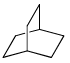has Plane of Symmetry so, show two signals.

QUESTION: 3

### What are the limits of detection of the following common analytical methods used with capillary separations: fluorescence, mass spectrometry, UV-Vis absorbance, and NMR, respectively, in mol:

Solution:
QUESTION: 4

Of the mo lecules CH4, CO2, benzene and H2, the ones that will absorb infrared radiation are:

Solution:
QUESTION: 5

Which one of the normal modes of ethylene is active in the infrared:

Solution:
QUESTION: 6

The vibrational stretching frequency of N2 can be determined using:

Solution:
QUESTION: 7

The number of IR active vibrational modes in ammonia is:

Solution:
QUESTION: 8

The number of vibrational degree of freedom in a gaseous mixture of 35Cl2O, 37Cl2O, and 35Cl, 37ClO is:

Solution:
QUESTION: 9

IR stretching frequencies of carbonyl groups in aldehydes and acid chlorides in cm–1 are:

Solution:
QUESTION: 10

Which among the following exhibits a carbonyl absorption band at 1770 cm–1:

Solution:

Cyclic Esters or Lactones show absorption band at 1770 cm–1

QUESTION: 11

Which one of the following exhibits rotational spectra:

Solution:
QUESTION: 12

The bond that gives the most intense band in the infrared spectrum for its stretching vibrations is:

Solution:
QUESTION: 13

An examinations of saturated hydrocarbons containing methyl group show asymmetrical (Vas) and symmetrical (Vs) stretching modes in the region of:

Solution:
QUESTION: 14

13C NMR spectrum of DMSO-d6 gives a signal at δ 39.7 ppm as a:

Solution:
QUESTION: 15

The molecule with the smallest rotational constant (in the microwave spectrum) among the following is:

Solution:
QUESTION: 16

Of the vibrational modes given below, the IR active mode(s) is (are):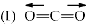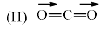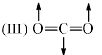Solution:
QUESTION: 17

Which of the fo llowing statements is/are true:

(I) HCl absorbs IR radiat ion
(II) CO2 absorbs IR radiat ion
(III) H atom absorbs IR radiation
(IV) H atom absorbs UV- vis and microwave radiation

Solution:
QUESTION: 18

N2 does not show pure vibrat ional spectral because:

Solution:
QUESTION: 19

How many normal modes does the CO2 molecule have; What if the C and the O atoms were constrained to move in one dimensional:

Solution:
QUESTION: 20

1H NMR spectrum of a mixture of benzene and acetonitrile shows two singlets of equal integration. The molar ratio of benzene: acetonitrile is:

Solution:

The signal intensity depends on number of equivalent hydrogen and acetonitrile have 3 hydrogen and benzene have six hydrogen.

Therefore the ratio is 1 : 2

QUESTION: 21

The absorption at λmax 279 nm (ε = 15) in the UV spectrum of acetone is due to:

Solution:
QUESTION: 22

1H NMR spectrum of an organic compound recorded on a 500 MHz spectrometer showed a quartet with line positions at 1759, 1753, 1747, 1741 Hz. Chemical shift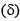and coupling constant (Hz) of the quartet are:

Solution:
QUESTION: 23

The correct statement in the context of NMR spectroscopy is:

Solution:
QUESTION: 24

The reaction between PI3, PSCl3 and zinc powder gives P3I5 as one of the products. The solution state 31P NMR spectrum of P3I5 shows a doublet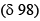and a triplet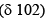. The correct structure of P3I5 is:

Solution:
QUESTION: 25

The compound that exhibits following spectral data is:

1H NMR: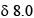(d, J = 12.3 Hz, 1H), 7.7 (d, J = 8.0 Hz, 2H), 6.8 (d, J = 8.0 Hz, 2H), 5.8 (d, J = 12.3 Hz, 1H), 3.8 (s, 3H), 3.0 (s, 6H) ppm

Solution:
QUESTION: 26

Number of signals present in the proton decoupled 13C NMR spectrum of the following compound is: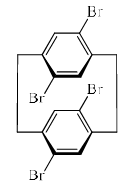Solution:

Due to Plane of Symmetry, only 4 signals are present.

QUESTION: 27

The structure of the compound having the following characteristics spectral data, is IR: 1690 cm–1.

1H-NMR: 1.30 (3H, t, J = 7.2 Hz); 2.41 (2H, q, J = 7.2 Hz); 2.32 (3H, s); 7.44 (1H, t, J = 7.0 Hz); 7.57 (1H, dt, J = 7.0, J = 7.0, 3.0 Hz); 7.77 (1H, t, J = 3.0 Hz); 7.90 (1H, dt, J = 7.0, 3.0 Hz); EI mass: m/z 119 (100%); 57 (80%)

Solution:
QUESTION: 28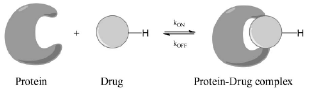NMR spectroscopy can be used to assay for drug binding to certain protein targets. One of the primary objective of any binding assay is the quantification of the free and the bound forms of a drug molecule at a certain concentration of the protein target. Let us assume that the fully bound drug exhibits a1H chemical shift of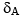while that of the free from resonated at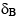for the same proton (see figure above). If the exchange timescale (i.e. proportional to kOFF as kON is diffusion limited) between the free form of the drug and its bound form is in microseconds, which of the following statements cannot be true assuming that the drug is only 50% bound with its protein target:

Solution:
QUESTION: 29

A compound with molecule formula C5H12O2 has strong infrared absorption at 3300 to 3400 cm–1. The 1H NMR spectrum showed three singlet at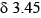and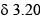ppm; with relative 3:2:1. Addition of D2O to the sample eliminates the lower field signal. The 13C NMR spectrum shows three signals all higher thanppm. Which of the following compounds best fits this data:

Solution:
QUESTION: 30

The optimized variation wave functions gives:

Solution: